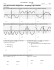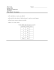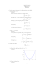# Higher Relationships and Calculus NAB Revision Pack

## Transcription

Higher Relationships and Calculus NAB Revision Pack
```Higher Relationships and Calculus
NAB Revision Pack
Assessment Standard 1.1
1.
Prove that x  2 is a factor of x 3  x 2  10x  8 and hence find the other factors.
2.
Prove that x  3 is a factor of x 3  x 2  9x  9 and hence fully factorise the expression.
3.
Factorise fully :
4.
Solve the equations :
5.
(a) x 3  21x  20
(b) 4x 3  8x 2  x  3
(a)
3x 3  7x 2  4  0
(b)
x 3  7x  6
(a)
Find the value of k which results in the equation k x 2  2kx  1  0 having equal roots,
given that k  0 ?
(b)
A quadratic equation is given as x 2  ( p  3) x  ( 1 4  3p)  0 .
For what values of p will the above equation have
i) equal roots
ii) no real roots?
Assessment Standard 1.2
6.
Solve each of the following equations :
(a) 2 sin 2x  1  0
,
for
0  x  360
(b) 2 cos 2x  3sin x  1  0 for 0  x  360
(c) cos x 
7.
1
2
for 0  x  360
sin 2x
(a)
Express 2 sin x  2 cos x in the form k cos ( x   ) where k > 0 and 0 <  < 90.
(b)
Hence solve the equation 2 sin x  2 cos x  2  81 for x where x > 40 .
Assessment Standard 1.3
8.
Differentiate each of the following functions with respect to the relevant variable :
(a)
f (x)  x3 (x  x2 )
(d)
f (t )  t 2 (t 2  t 2 ) (e)
(g)
x5  2x 2
g(x) 
x4
1
(b)
3
(h)
g ( x )  3x 2 
g ( p) 
1
x3
1  13
2
(p  p 3)
p
5v  2
f (v ) 
v
1
1

x
3x 2
(c)
h (x) 
(f)
h (u)  u 
(i)
1  t2  t 2 
h (t ) 


t t 
1
2 u
3
9.
10.
11.
The amount of pressure P (pounds per square inch) within a cylinder varies with time t
(milliseconds) according to the formula P (t )  400t  50t 2 .
(a)
Calculate the rate of change of P when t  2 .
(b)
Calculate how fast P is changing when t  4 .
(c)
How is the rate of P changing when t  5 ? Comment
( x  1)
dx
x2
(a)
Find the equation of the tangent to the curve with equation y  3x 2  2 x at
the point where x  1 .
(b)
Find the equation of the tangent to the curve y 
1
 x at the point where x 
x
Differentiate the following functions with respect to x :
(a)
f(x) = 3sinx
(b)
f(x) = 9cosx
(c)
( 3x  x 2 ) 2 dx
(c)
f(x) = 2sinx – 5cosx
Assessment Standard 1.4
12.
Find :
(a)
13.
(b)
3cosx dx
(b)
Find :
(a)
14.

( 9 x 2  6x ) dx




( x  1)
dx
x2

7sinx dx
(c)
Evaluate each of the following definite integrals :
(a)

4
1
x2  2 x
dx
x
(b)

1
1


1
1
3v3  v  3 dv
v
2cosθ – sinθ dθ
1
2
.
```

### pdf file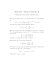### Math 120 Sample questions for Test 2, with answers### AS Entrance Examination Sample Paper Mathematics Instructions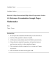### Sample Solutions to Quiz 3 for MATH3270A − y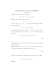### Test4-Review - FIU Faculty Websites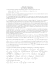### MATH 307: Problem Set #6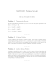### Calculus I Final, Sample### Worksheet 6 Chain rule and implicit differentiation 1 Chain rule 2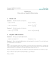### Review Sheet for Second Midterm Mathematics 1300, Calculus 1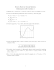### 1.6 Exam advice and sample questions for Chapter 1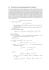### Time : 120 Minutes Max. Marks : 60 GENERAL INSTRUCTIONS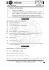### Homework 33: Stokes Theorem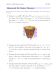### Document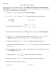### Chapter 6 Problems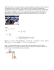### Departmental Practice Final Version 2 15400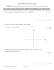### Midterm I Key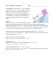### Problem Set # 5 / MATH 20400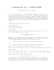### Mathematics - Paper - II (Set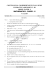### Key for Exam 3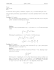### File - Pre